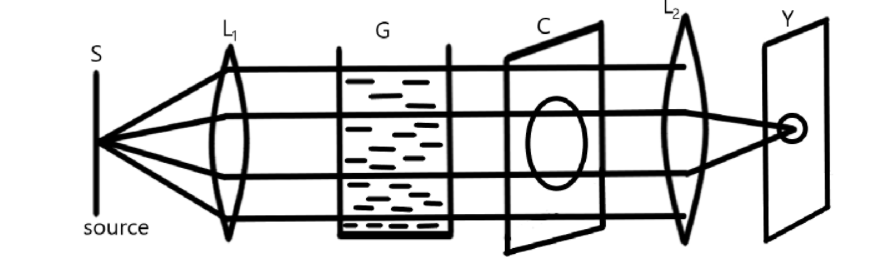# Draw diagram of an experimental arrangement for observing scattering of light incolloidal solution. Name the two chemicals used in this activity.Verified
198.9k+ views
Hint:The arrangement used for the scattering of light consists of a source of light placed in front of the converging lens. A transparent glass tank, a cardboard that contains hole, a converging lens that converges the light on the screen. The chemicals used in this activity will be used for producing Tyndall effect.

COMPLETE STEP BY STEP ANSWER:
The diagram showing the scattering of light in the colloidal solution is given byHere, $S$ is the source of light from the rays of light are emerging, ${L_1}$ and ${L_2}$ are the convex lenses, $G$ is the transparent glass surface that contains colloidal solution, $C$ is the cardboard that contains hole and $Y$ is the screen on which we get an image.

As shown in the figure, a source $S$ is placed in front of a convex lens that allows the rays of light to fall in the transparent glass tank that contains the colloidal solution that enables the Tyndall effect of the rays of light. After the Tyndall effect, these rays of light fall on the cardboard that consists of hole.

Some of the rays of light pass through the holes and fall on the converging lens. This converging lens will converge the rays of light on the screen that forms the image on the screen.

The solution in the tank contains $2l$ of the water in which $1\,ml$ to $2\,ml$ of sulphuric acid
$\left( {{H_2}S{O_4}} \right)$ is added.

Therefore, the two chemicals used in this activity are water and sulphuric acid.

NOTE:The above arrangement is used to show the scattering of light. This arrangement can also be used to determine why the sky appears blue and why sunset appears red. When the wavelength of the light becomes lower, the color will be blue. On the other hand, when the wavelength of the light will be greater, the color will be red.